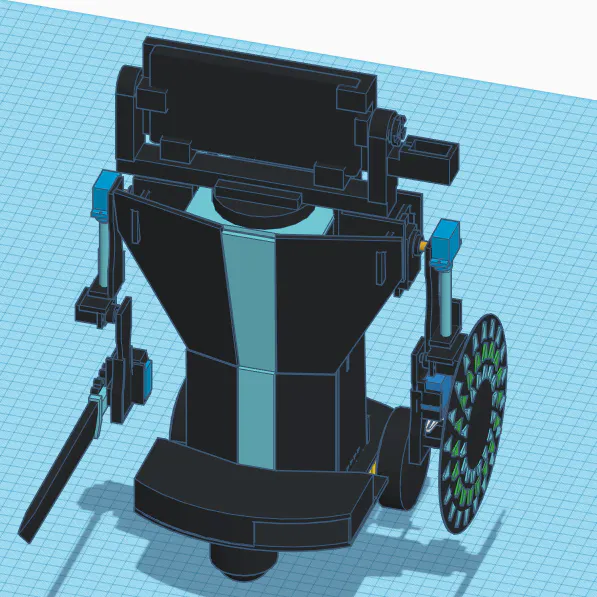# Mini Me, the Mini Humanoid Robot

Imagin being able to controll with your movements a mini version of yourself, well that's what this project is all about.

IntermediateWork in progress570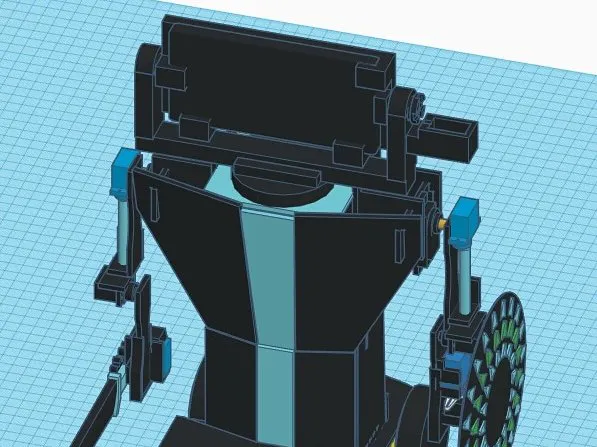## Things used in this project

### Hardware components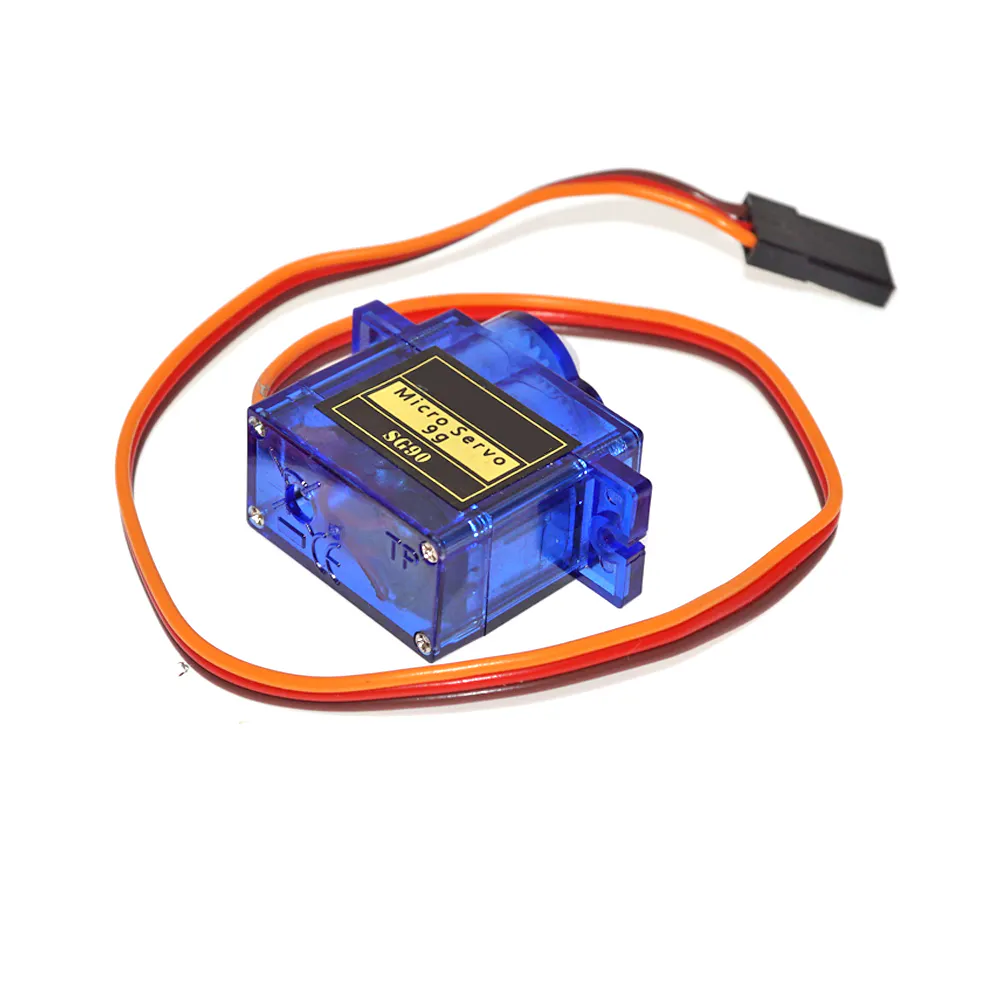SG90 Micro-servo motor
×10
 MG90 Servo Motor
×4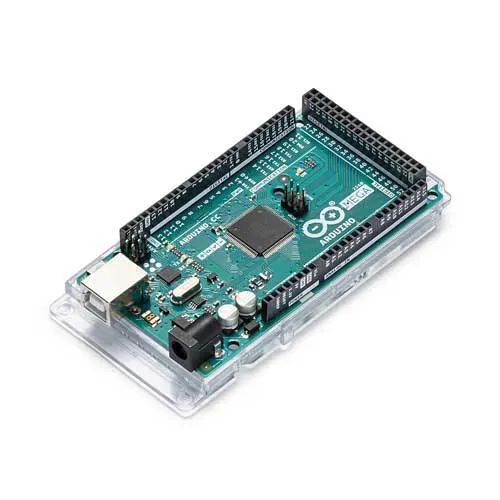Arduino Mega 2560
×1

### Software apps and online servicesArduino IDE

### Hand tools and fabrication machines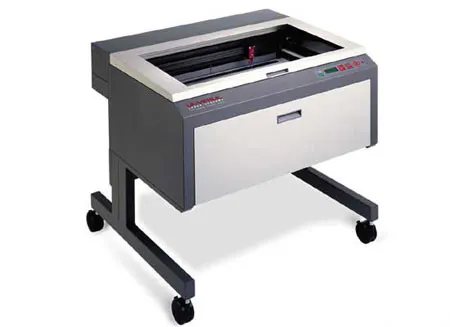Laser cutter (generic)

## Code

### Testing code

C/C++
This is the testing code i've beeing using for testing the strength of the servos
```#include <Servo.h>

int pos;
Servo pinza;
Servo muneca;
Servo codo;
Servo codoG;
Servo hombro;

void setup() {
pinza.attach(2);
muneca.attach(3);
codo.attach(4);
codoG.attach(5);
hombro.attach(6);

muneca.write(100);
codo.write(30);
codoG.write(100);
hombro.write(30);

}

void loop() {
//pinza
for (pos = 130; pos <= 180; pos += 1) { // goes from 0 degrees to 180 degrees
// in steps of 1 degree
pinza.write(pos);              // tell servo to go to position in variable 'pos'
delay(15);                       // waits 15ms for the servo to reach the position
}
for (pos = 180; pos >= 130; pos -= 1) { // goes from 180 degrees to 0 degrees
pinza.write(pos);              // tell servo to go to position in variable 'pos'
delay(15);                       // waits 15ms for the servo to reach the position
}

//muñeca
for (pos = 0; pos <= 180; pos += 1) { // goes from 0 degrees to 180 degrees
// in steps of 1 degree
muneca.write(pos);              // tell servo to go to position in variable 'pos'
delay(15);                       // waits 15ms for the servo to reach the position
}
for (pos = 180; pos >= 0; pos -= 1) { // goes from 180 degrees to 0 degrees
muneca.write(pos);              // tell servo to go to position in variable 'pos'
delay(15);                       // waits 15ms for the servo to reach the position
}
muneca.write(100);

//codo
for (pos = 0; pos <= 130; pos += 1) { // goes from 0 degrees to 180 degrees
// in steps of 1 degree
codo.write(pos);              // tell servo to go to position in variable 'pos'
delay(15);                       // waits 15ms for the servo to reach the position
}
for (pos = 130; pos >= 0; pos -= 1) { // goes from 180 degrees to 0 degrees
codo.write(pos);              // tell servo to go to position in variable 'pos'
delay(15);                       // waits 15ms for the servo to reach the position
}
codo.write(30);

//codoG
for (pos = 0; pos <= 180; pos += 1) { // goes from 0 degrees to 180 degrees
// in steps of 1 degree
codoG.write(pos);              // tell servo to go to position in variable 'pos'
delay(15);                       // waits 15ms for the servo to reach the position
}
for (pos = 180; pos >= 0; pos -= 1) { // goes from 180 degrees to 0 degrees
codoG.write(pos);              // tell servo to go to position in variable 'pos'
delay(15);                       // waits 15ms for the servo to reach the position
}
codoG.write(100);

//hombro
for (pos = 0; pos <= 180; pos += 1) { // goes from 0 degrees to 180 degrees
// in steps of 1 degree
hombro.write(pos);              // tell servo to go to position in variable 'pos'
delay(15);                       // waits 15ms for the servo to reach the position
}
for (pos = 180; pos >= 0; pos -= 1) { // goes from 180 degrees to 0 degrees
hombro.write(pos);              // tell servo to go to position in variable 'pos'
delay(15);                       // waits 15ms for the servo to reach the position
}

hombro.write(30);
}
```

### Code for the exoskeleton

C/C++
This is the code that goes into the nano in the exoskeleton. It uses the EasyTransfer Library, o make ure you have it istalled.
```#include <EasyTransfer.h>

//create object
EasyTransfer ET;

struct SEND_DATA_STRUCTURE{
//put your variable definitions here for the data you want to send
//THIS MUST BE EXACTLY THE SAME ON THE OTHER ARDUINO
int16_t shoulderPot;
int16_t elbowRPot;
int16_t elbowPot;
};

//give a name to the group of data
SEND_DATA_STRUCTURE mydata;

void setup() {
Serial.begin(9600);

//start the library, pass in the data details and the name of the serial port. Can be Serial, Serial1, Serial2, etc.
ET.begin(details(mydata), &Serial);

}

void loop() {

ET.sendData();

/*
Serial.print(mydata.shoulderPot);
Serial.print(" , ");
Serial.print(mydata.elbowRPot);
Serial.print(" , ");
Serial.println(mydata.elbowPot);
delay(100);
*/
delay(50);
}
```

### Exoskeleton code for the robot

C/C++
This code goe on to the Mega in the robot. It reads the values sent by the other arduino and map it to use them to move the servos. It ues the EasyTranfer library for sending and reciving data, so make sure you have it istalled.
```#include <EasyTransfer.h>

//create object
EasyTransfer ET;

//put your variable definitions here for the data you want to receive
//THIS MUST BE EXACTLY THE SAME ON THE OTHER ARDUINO
int16_t pause;
};

//give a name to the group of data

void setup(){
Serial.begin(9600);
//start the library, pass in the data details and the name of the serial port. Can be Serial, Serial1, Serial2, etc.
ET.begin(details(mydata), &Serial);

pinMode(13, OUTPUT);

}

void loop(){
//check and see if a data packet has come in.
//this is how you access the variables. [name of the group].[variable name]
//since we have data, we will blink it out.
for(int i = mydata.blinks; i>0; i--){
digitalWrite(13, HIGH);
delay(mydata.pause * 100);
digitalWrite(13, LOW);
delay(mydata.pause * 100);
}
}

//you should make this delay shorter then your transmit delay or else messages could be lost
delay(250);
}
```

## Credits

### CoolRobotsAndStuff

1 project • 0 followers